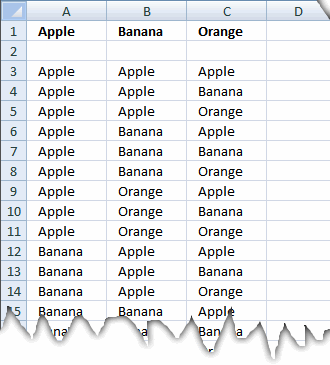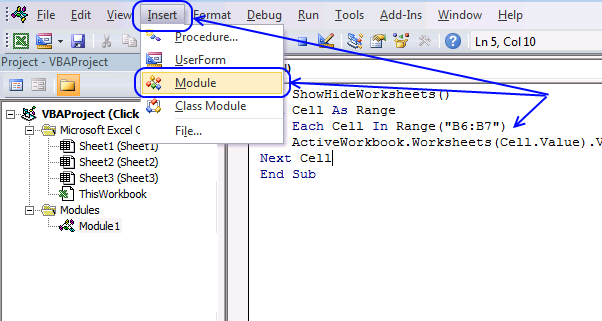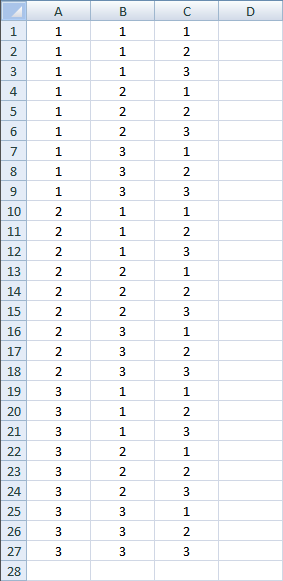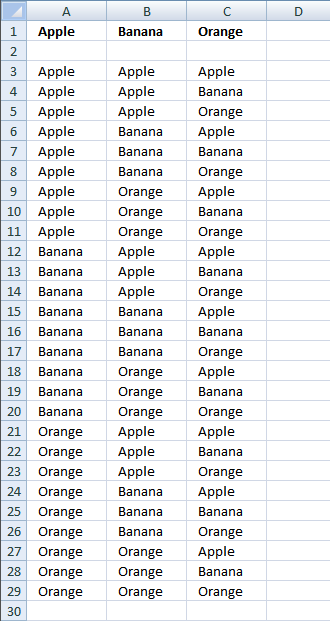Author: Oscar Cronquist Article last updated on January 08, 2019This blog post demonstrates a custom function (UDF) that creates permutations. Repetition is allowed. The custom function lets you specify the number of items to use and it will return an array of numbers.

Array formula:

=ListPermut(3)

To enter an array formula, type the formula in a cell then press and hold CTRL + SHIFT simultaneously, now press Enter once. Release all keys.

The formula bar now shows the formula with a beginning and ending curly bracket telling you that you entered the formula successfully. Don't enter the curly brackets yourself.

### Vba

```Function ListPermut(num As Integer)

'Permutations with repetition

Dim c As Long, r As Long, p As Long
Dim rng() As Long
p = num ^ num
ReDim rng(1 To p, 1 To num)

For c = 1 To num
rng(1, c) = 1
Next c

For r = 2 To p
For c = num To 1 Step -1
If c = num Then
rng(r, c) = rng(r - 1, c) + 1
ElseIf rng(r, c) = 0 Then
rng(r, c) = rng(r - 1, c)
End If
If rng(r, c) = num + 1 Then
rng(r, c) = 1
rng(r, c - 1) = rng(r - 1, c - 1) + 1
End If
Next c
Next r

ListPermut = rng

End Function
```The image above shows a macro that is not used in this article, it is only there to show you where to paste the code.

1. Press Alt-F11 to open visual basic editor.
2. Press with left mouse button on Module on the Insert menu.
3. Copy the above user defined function.
4. Paste it to the code module.
5. Exit visual basic editor.
6. Select sheet1.
7. Select cell range A1:C27.
8. Type =ListPermut(3) in formula bar and press CTRL+SHIFT+ENTER (Array formula).
If you don't know how to enter an array formula then read the detailed instructions below the image.
Note: Save your workbook with the file extension *.xlsm (Macro-enabled workbook) in order to keep the custom function next time you open the workbook.Example,Array formula in A3:C29:

=INDEX(\$A\$1:\$C\$1, 1, ListPermut(3)) + CTRL + SHIFT + ENTER

### Get the Excel fileListPermut.xls